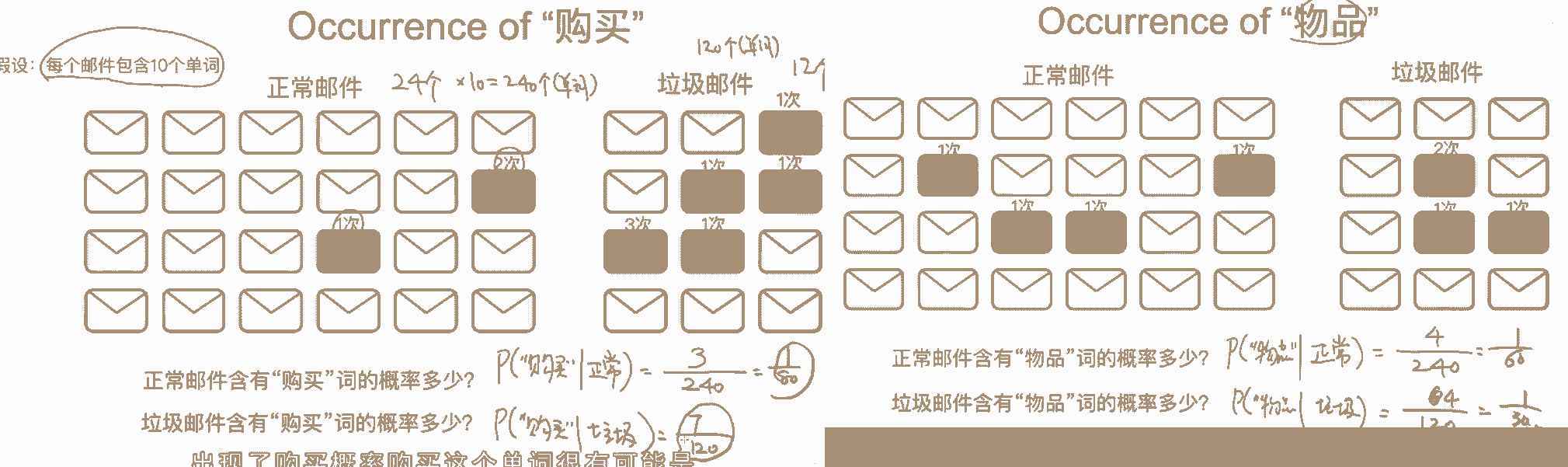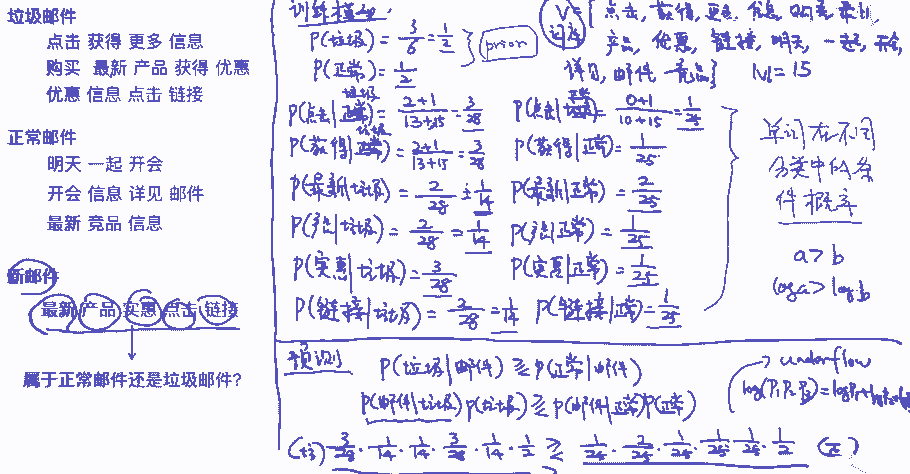# 塞进裤子ヾ(≧O≦)〃嗷~

0%

## 任务：

$P(y|X) = \frac{P(X|y)P(y)}{P(X)}=\frac{P(x_1,x_2,x_3|y)P(y)}{P(X)}$

$\frac{P(x_1,x_2,x_3|y)P(y)}{P(X)} = \frac{P(x_1|y)P(x_2|y)P(x_3|y)P(y)}{P(X)}$

## 算法：

$P(c_i|W) = \frac{P(W|c_i)P(c_i)}{P(W)}$

$W$为test文本单词构成的向量，$c_i$表示属于类别i

$P(c_i)$ 表示属于ci的文档出现的概率=count（属于ci的文档）/count（总文档数）
$P（W|ci）= p(w0 | ci)p(w1 | ci)p(w2 | ci)…p(wn | ci)$ 朴素，每个特征相互独立
P（w0|ci）wo在ci的文档中出现的次数/ w0在所有文档出现的总次数 错
P（w0|ci）wo在ci的文档中出现的次数/ ci的文档中出现的单词总数 对

• 1 建立词汇表
• 2.计算$P(c_i)$和$P(W|c_i)$
• 3 test时，找到每个test word所对应的$P(w_j|c_i)$,计算P(c_i|W) =$P(c_i)$和$P(W|c_i)$,找到概率最大的类别。

• 为防止分子太小，使得概率为0，加入$log(P)$

• 在利用贝叶斯分类器对文档进行分类时，要计算多个概率的乘积以获得文档属于某个类别的概率，即计算 p(w0|1) * p(w1|1) * p(w2|1)。如果其中一个概率值为 0，那么最后的乘积也为 0。为降低这种影响，可以将所有词的出现数初始化为 1，并将分母初始化为 2 （取1 或 2 的目的主要是为了保证分子和分母不为0，大家可以根据业务需求进行更改）。

## 例子：

$P(正常|内容) = \frac{P(内容|正常)P(正常)}{P(内容)}$

$P(垃圾|内容) = \frac{P(内容|垃圾)P(垃圾)}{P(内容)}$

$P(正常)$ 为先验概率，即正常邮件在所有邮件中的占比， $P(垃圾)$同理

$P(内容|正常) = p(购买，物品，不是，广告|正常) = P（购买|正常）P（物品|正常）P（不是|正常）P（广告|正常）$

$P(购买|正常) = \frac{正常邮件中出现的购买单词的次数}{正常邮件中的所有单词的总数(含重复)}$## 例子2：